Scanner - Production function(2008-2018)

# Scanner - Production function(2008-2018) - Notes | Study Economics Class 11 - Commerce

 1 Crore+ students have signed up on EduRev. Have you?

NCERT Questions

(Q1) Explain the concept of a production function.

(Q2) What is the total product, marginal product and average product of an input?

(Q3) Explain the relationship between the marginal products and the total product of an input

(Q4) Explain the concepts of short run and the long run.

(Q5) What is the law of diminishing marginal product?

(Q6) Find AP, TP, MP as the case may be (assume total product of labor is zero at zero levels of
employment)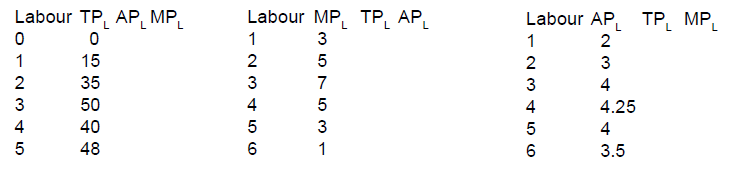(Q7) Let the production function of a firm be Q = 5 L1/2 K1/2 Find out the maximum output a firm can produce with 100 units of L and 100 units of K

(Q8) Q = 2L 2K2. Find out the maximum output the firm can produce with 5 units of L and 2 units of K? What is the maximum possible output that the firm can produce with zero units of Land 10 units of K

(Q9) What is the maximum possible output that the firm can produce with zero units of Land 10 units of K when its P. F is Q = 5L and 2K

(Q10) List any three input used in production?

(Q11) How is the total physical product derived from the marginal physical product schedule?

(Q12) What will you say about MPP of a factor when TPP is falling?

(Q13) What is the general shape of APP and MPP curve?

(Q14 ) How does TPP changes with a change in MPP of an input?

C. B. S. E. Question’s

(Q1) Identify the three phases of the law of Variable Proportion from the following and also give a reason behind each phase :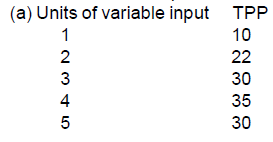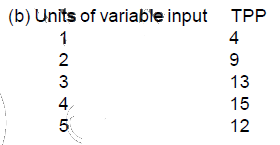(Q2) Define (a) Production function (b) Marginal Physical Product

(Q3) What is meant by a return to a factor? What leads to increasing returns to a factor?

(Q5) Explain the law of variable proportion with the help of TP and MP curves?

OR

Explain the law of variable proportion in terms of the behavior of total physical product with the help of  diagram

OR

Explain the three stages of production, when one-factor input is variable.

(Q6) Explain the law of variable proportion and the reasons behind it? OR Why does the MP declines with further employment of factor?

Sample Paper and C.B.S.E 2008 & 2009 Question’s

(Q1) Define production function (1)

(Q2) Explain the reasons for increasing returns to a factor (3)

(Q3) Explain the likely behavior of Total Product and Marginal Product when only one input is increased while all other inputs are kept unchanged.

(Q4) Giving reasons, state whether the following statements are true or false:

(i) When there are diminishing returns to a factor, marginal product and total product both always diminish.
(ii) TP will increase only when MP increases
(iii) Increase in TP always indicates that there is an increasing return to a factor

CBSE 2010 + Sample Paper Questions

(Q1) Total product always increases whether there is increasing returns or diminishing returns to a factor. State True or False (2M)

(Q2) Explain the law of returns to a factor with the help of total product and marginal product schedule. (6M)

(Q3) What change will take place in marginal product, when total product increases at a diminishing rate? (1 M)

(Q4) Explain the likely behavior of Total Product and Marginal Product when for increasing production only one input is increased while all other inputs are kept constant. (6 M)

CBSE 2011 & CBSE 2012

(Q1) Define production function. (1 mark)

(Q2) What does the Law of Variable Proportions show? State the behavior of the marginal product according to this law (4 M)

Sample Paper 2014-15 & 15-16 & 16-17

(Q1) Complete the following schedule: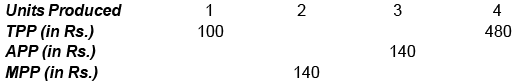(Q2) State the behavior of Marginal Physical Product, under Returns to a Factor. (3)

(a) MPP initially rises to its maximum

(b)  MPP then decreases (stays positive) to become zero

(c) MPP becomes negative

(Q3) State true or false:  Average product rises only where marginal product rises.
False: Average product rises as long as the marginal product is greater than average product. Here marginal product could be rising or falling.

C.B.S.E & Sample Paper 2013

(Q1) Giving reasons, state whether the following statements are true or false :( 2M each )

(i) The average product will increase only when marginal product increases.

(ii) Under diminishing returns to a factor, the total product continues to increase till marginal
the product reaches Zero.

False. The average product will increase only when marginal product is greater than average product whether MP is rising or falling.

True Under diminishing returns, MP falls. TP increases till MR is positive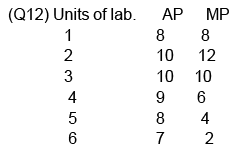C.B.S.E Paper 2014

(Q1) Define marginal product.  (1M)

Ans:  It is the addition to the total product when one more unit of the variable input is increased.

Ans: Returns to a factor refers to change in output when only one input is changed, other inputs remaining unchanged.

(Q3) Giving reasons, explain the ‘Law of Variable Proportions’. (4 M)

(Q4) Explain the behavior of MP in the law of variable proportion. Also, explain its causes?

Sample Paper 2015

(a) Average product rises only where marginal product rises.
C.B.S.E Paper 2015

(Q1) What are the different phases in the Law of Variable Proportions in terms of marginal product? Give the reasons behind each phase. Use the diagram. (6 M)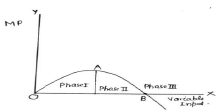(Q2) State whether the following statements are true or false, with reasons. (a) When the total product is constant, the average product will fall.[Delhi (C)]

(Q3) Explain the changes that take place in total product and marginal product under increasing returns to a factor.[AI (C)]

(Q4) Explain the changes that take place in total product and marginal product under diminishing returns to a factor.[AI (C)]

(Q5) Complete the following schedule: [SP (2+4M)]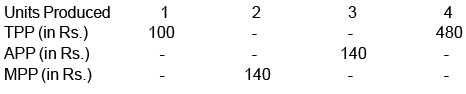CBSE 2016

(Q1) Define marginal product. State the behavior of marginal product when only one input is increased and other inputs are held constant.

(Q2) Define production function. Distinguish between short run and long run production functions. (4)

Ans: Production function refers to a mathematical relation between physical input and physical output. (1)

Short run production function refers to a situation when output is increased by changing only one input while keeping other inputs unchanged. (11/2)

Long run production function refers to a situation when output is increased by increasing all the inputs simultaneously and in the same proportion. (11/2)

(Q3) What type of production function is this in which only one input is increased and others kept constant? State the behavior of total product in this production function.

Ans: Short run production function. · Initially TP increases at an increasing rate. - After a certain level of output, TP increases at a decreasing rate. - Ultimately TP falls.

CBSE 2017

(Q1)State the relation between marginal product and average product. Show this relation in a diagram; 0r

State the relation between marginal product and average product. Show this relation in a schedule.

(Q2) Give the behavior of marginal product and total product as more and more units of only one input are employed while keeping other inputs as constant.        , or

State different phases of the law of variable proportions on the basis of total product. Use the diagram.

According to the Law of Variable Proportions – as only one input is increased, others remaining unchanged, the total product increases at an increasing rate, then at a decreasing rate and ultimately falls.

Phase I: In the first phase, TP increases at an increasing rate up to point A. 1

Phase II: In the second phase, TP increases at a diminishing rate between point A & B.   1

Phase III: In the third phase, TP starts falling beyond point B.   1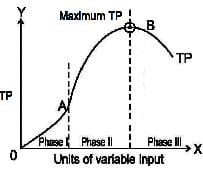(Q3) Identify the three phases of the law of variable proportions. Give reasons.

Variable input  (units)        Total product(units)

1                                                3

2                                                7

3                                               10

4                                                12

5                                                11

(Q4)  Identify the three phases of the law of Variable Proportion from the following and also give a reason behind each phase :

Units of variable input      TPP

1                                   10

2                                   22

3                                   30

4                                   35

5                                   30

CBSE 2018

(Q1) When the Average Product (AP) is maximum, the Marginal Product (MP) is :     (1M)(a) Equal to AP

(b) Less than AP

(c) More than AP

(d) Can be any one of the above

Ans: (a)

The document Scanner - Production function(2008-2018) - Notes | Study Economics Class 11 - Commerce is a part of the Commerce Course Economics Class 11.
All you need of Commerce at this link: Commerce

## Economics Class 11

201 videos|199 docs|64 tests

## Economics Class 11

201 videos|199 docs|64 tests

Track your progress, build streaks, highlight & save important lessons and more!

,

,

,

,

,

,

,

,

,

,

,

,

,

,

,

,

,

,

,

,

,

;